A tube of length L is filled completely with an incompressible liquid of mass M and closed at both ends. The tube is then rotated in a horizontal plane about one of its ends with a uniform angular velocity $\omega$. The force exerted by liquid at the other end is

1.  $\frac{{\mathrm{M\omega }}^{2}\mathrm{L}}{2}$

2.  ${\mathrm{ML\omega }}^{2}$

3.  $\frac{{\mathrm{M\omega }}^{2}\mathrm{L}}{4}$

4.  $\frac{M{L}^{2}{\omega }^{2}}{2}$

Subtopic:  Pressure |
To view explanation, please take trial in the course below.
NEET 2022 - Target Batch - Aryan Raj Singh

Difficulty Level:

The radius of a soap bubble is increased from R to 2 R. Work done in this process (T = surface tension) is

1.

2.

3.

4.

Subtopic:  Surface Tension |
To view explanation, please take trial in the course below.
NEET 2022 - Target Batch - Aryan Raj Singh
To view explanation, please take trial in the course below.
NEET 2022 - Target Batch - Aryan Raj Singh

Difficulty Level:

The flow rate from a tap of diameter 1.25 cm is 3 lit/min. The coefficient of viscosity of water is ${10}^{-3}$ Pas. The nature of flow is :

1. Turbulent

2. Laminar

3. Neither laminar nor turbulent

Subtopic:  Types of Flows |
To view explanation, please take trial in the course below.
NEET 2022 - Target Batch - Aryan Raj Singh
To view explanation, please take trial in the course below.
NEET 2022 - Target Batch - Aryan Raj Singh

Difficulty Level:

A small spherical solid ball is dropped in a viscous liquid. Its journey in the liquid is best described in the figure by :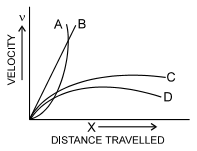1.  Curve A

2.  Curve B

3.  Curve C

4.  Curve D

Subtopic:  Stokes' Law |
To view explanation, please take trial in the course below.
NEET 2022 - Target Batch - Aryan Raj Singh
To view explanation, please take trial in the course below.
NEET 2022 - Target Batch - Aryan Raj Singh

Difficulty Level:

Water flows through a frictionless duct with a cross-section varying as shown in the figure. Pressure p at points along the axis is represented by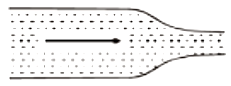1.2.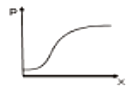3.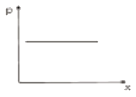4.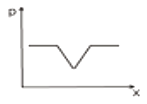Subtopic:  Equation of Continuity |
To view explanation, please take trial in the course below.
NEET 2022 - Target Batch - Aryan Raj Singh
To view explanation, please take trial in the course below.
NEET 2022 - Target Batch - Aryan Raj Singh

Difficulty Level:

The area of cross-section of the wider tube shown in the figure is  If a mass of 12 kg is placed on the massless piston, the difference in heights h in the level of water in the two tubes is :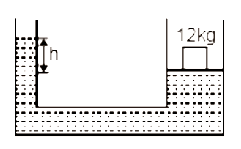1.  10 cm

2.  6 cm

3.  15 cm

4.  2 cm

Subtopic:  Pressure |
To view explanation, please take trial in the course below.
NEET 2022 - Target Batch - Aryan Raj Singh
To view explanation, please take trial in the course below.
NEET 2022 - Target Batch - Aryan Raj Singh

Difficulty Level:

Equation of continuity based on :

1.  Conservation of mass

2.  Conservation of energy

3.  Conservation of angular momentum

4.  None of these

Subtopic:  Equation of Continuity |
To view explanation, please take trial in the course below.
NEET 2022 - Target Batch - Aryan Raj Singh
To view explanation, please take trial in the course below.
NEET 2022 - Target Batch - Aryan Raj Singh

Difficulty Level:

The cylindrical tube of a spray pump has radius R, one end of which has n fine holes, each of radius r. If the speed of the liquid in the tube is v, the speed of the ejection of the liquid through the holes is

1. $\frac{{\mathrm{vR}}^{2}}{{\mathrm{n}}^{2}{\mathrm{r}}^{2}}$

2. $\frac{{\mathrm{vR}}^{2}}{{\mathrm{nr}}^{2}}$

3. $\frac{{\mathrm{vR}}^{2}}{{\mathrm{n}}^{2}{\mathrm{r}}^{2}}$

4. $\frac{{\mathrm{v}}^{2}\mathrm{R}}{\mathrm{nr}}$

Subtopic:  Equation of Continuity |
To view explanation, please take trial in the course below.
NEET 2022 - Target Batch - Aryan Raj Singh

Difficulty Level:

The weight of an aeroplane flying in the air is balanced by

(1) Vertical component of the thrust created by air currents striking the lower surface of the wings

(2) Force due to reaction of gases ejected by the revolving propeller

(3) Upthrust of the air which will be equal to the weight of the air having the same volume as the plane

(4) Force due to the pressure difference between the upper and lower surfaces of the wings created by different air speeds on the surfaces

Subtopic:  Bernoulli's Theorem |
To view explanation, please take trial in the course below.
NEET 2022 - Target Batch - Aryan Raj Singh
To view explanation, please take trial in the course below.
NEET 2022 - Target Batch - Aryan Raj Singh

Difficulty Level:

If there were a smaller gravitational effect, which of the following forces do you think would alter in some respect?

(1)  Viscous forces

(2)   Archimedes uplift

(3)   Electrostatic force

(4)   None of the above

Subtopic:  Archimedes' Principle |
To view explanation, please take trial in the course below.
NEET 2022 - Target Batch - Aryan Raj Singh
To view explanation, please take trial in the course below.
NEET 2022 - Target Batch - Aryan Raj Singh

Difficulty Level: